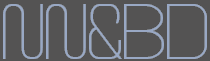# 基于双向BiLstm神经网络的中文分词详解及源码

## 1 标注序列

人/b  们/e  常/s  说/s  生/b  活/e  是/s  一/s  部/s  教/b  科/m  书/e


## 2 训练网络

RNN的意思是，为了预测最后的结果，我先用第一个词预测，当然，只用第一个预测的预测结果肯定不精确，我把这个结果作为特征，跟第二词一起，来预测结果；接着，我用这个新的预测结果结合第三词，来作新的预测；然后重复这个过程；直到最后一个词。这样，如果输入有n个词，那么我们事实上对结果作了n次预测，给出了n个预测序列。整个过程中，模型共享一组参数。因此，RNN降低了模型的参数数目，防止了过拟合，同时，它生来就是为处理序列问题而设计的，因此，特别适合处理序列问题。循环神经网络原理见下图：LSTM对RNN做了改进，使得能够捕捉更长距离的信息。但是不管是LSTM还是RNN，都有一个问题，它是从左往右推进的，因此后面的词会比前面的词更重要，但是对于分词这个任务来说是不妥的，因为句子各个字应该是平权的。因此出现了双向LSTM，它从左到右做一次LSTM，然后从右到左做一次LSTM，然后把两次结果组合起来。## 4 keras代码讲解embedded = Embedding(len(chars) + 1, word_size, input_length=maxlen, mask_zero=True)(sequence)并将不足的补零。

from keras.layers import Dense, Embedding, LSTM, TimeDistributed, Input, Bidirectional
from keras.models import Model

def create_model(maxlen, chars, word_size):
"""

:param maxlen:
:param chars:
:param word_size:
:return:
"""
sequence = Input(shape=(maxlen,), dtype='int32')
embedded = Embedding(len(chars) + 1, word_size, input_length=maxlen, mask_zero=True)(sequence)
blstm = Bidirectional(LSTM(64, return_sequences=True), merge_mode='sum')(embedded)
output = TimeDistributed(Dense(5, activation='softmax'))(blstm)
model = Model(input=sequence, output=output)
return model



# -*- coding:utf-8 -*-

import re
import numpy as np
import pandas as pd

# 设计模型
word_size = 128
maxlen = 32

with open('data/msr_train.txt', 'rb') as inp:
s = texts.split('\r\n')  # 根据换行切分

def clean(s):  # 整理一下数据，有些不规范的地方
if u'“/s' not in s:
return s.replace(u' ”/s', '')
elif u'”/s' not in s:
return s.replace(u'“/s ', '')
elif u'‘/s' not in s:
return s.replace(u' ’/s', '')
elif u'’/s' not in s:
return s.replace(u'‘/s ', '')
else:
return s

s = u''.join(map(clean, s))
s = re.split(u'[，。！？、]/[bems]', s)

data = []  # 生成训练样本
label = []

def get_xy(s):
s = re.findall('(.)/(.)', s)
if s:
s = np.array(s)
return list(s[:, 0]), list(s[:, 1])

for i in s:
x = get_xy(i)
if x:
data.append(x)
label.append(x)

d = pd.DataFrame(index=range(len(data)))
d['data'] = data
d['label'] = label
d = d[d['data'].apply(len) <= maxlen]
d.index = range(len(d))
tag = pd.Series({'s': 0, 'b': 1, 'm': 2, 'e': 3, 'x': 4})

chars = []  # 统计所有字，跟每个字编号
for i in data:
chars.extend(i)

chars = pd.Series(chars).value_counts()
chars[:] = range(1, len(chars) + 1)

# 保存数据
import pickle

with open('model/chars.pkl', 'wb') as outp:
pickle.dump(chars, outp)
print('** Finished saving the data.')

# 生成适合模型输入的格式
from keras.utils import np_utils

d['x'] = d['data'].apply(lambda x: np.array(list(chars[x]) +  * (maxlen - len(x))))

def trans_one(x):
_ = map(lambda y: np_utils.to_categorical(y, 5), tag[x].reshape((-1, 1)))
_ = list(_)
_.extend([np.array([[0, 0, 0, 0, 1]])] * (maxlen - len(x)))
return np.array(_)

d['y'] = d['label'].apply(trans_one)

import lstm_model

model = lstm_model.create_model(maxlen, chars, word_size)

batch_size = 1024
history = model.fit(np.array(list(d['x'])), np.array(list(d['y'])).reshape((-1, maxlen, 5)), batch_size=batch_size,
nb_epoch=20, verbose=2)
model.save('model/model.h5')1080显卡训练每次需要耗时44s,训练20个epoch后精度达到95%

import pickle
import lstm_model
import pandas as pd

with open('model/chars.pkl', 'rb') as inp:
word_size = 128
maxlen = 32

model = lstm_model.create_model(maxlen, chars, word_size)

import re
import numpy as np

# 转移概率，单纯用了等概率
zy = {'be': 0.5,
'bm': 0.5,
'eb': 0.5,
'es': 0.5,
'me': 0.5,
'mm': 0.5,
'sb': 0.5,
'ss': 0.5
}

zy = {i: np.log(zy[i]) for i in zy.keys()}

def viterbi(nodes):
paths = {'b': nodes['b'], 's': nodes['s']}  # 第一层，只有两个节点
for layer in range(1, len(nodes)):  # 后面的每一层
paths_ = paths.copy()  # 先保存上一层的路径
# node_now 为本层节点， node_last 为上层节点
paths = {}  # 清空 path
for node_now in nodes[layer].keys():
# 对于本层的每个节点，找出最短路径
sub_paths = {}
# 上一层的每个节点到本层节点的连接
for path_last in paths_.keys():
if path_last[-1] + node_now in zy.keys():  # 若转移概率不为 0
sub_paths[path_last + node_now] = paths_[path_last] + nodes[layer][node_now] + zy[
path_last[-1] + node_now]
# 最短路径,即概率最大的那个
sr_subpaths = pd.Series(sub_paths)
sr_subpaths = sr_subpaths.sort_values()  # 升序排序
node_subpath = sr_subpaths.index[-1]  # 最短路径
node_value = sr_subpaths[-1]  # 最短路径对应的值
# 把 node_now 的最短路径添加到 paths 中
paths[node_subpath] = node_value
# 所有层求完后，找出最后一层中各个节点的路径最短的路径
sr_paths = pd.Series(paths)
sr_paths = sr_paths.sort_values()  # 按照升序排序
return sr_paths.index[-1]  # 返回最短路径（概率值最大的路径）

def simple_cut(s):
if s:
r = model.predict(np.array([list(chars[list(s)].fillna(0).astype(int)) +  * (maxlen - len(s))]),
verbose=False)[
0][:len(s)]
r = np.log(r)
print(r)
nodes = [dict(zip(['s', 'b', 'm', 'e'], i[:4])) for i in r]
t = viterbi(nodes)
words = []
for i in range(len(s)):
if t[i] in ['s', 'b']:
words.append(s[i])
else:
words[-1] += s[i]
return words
else:
return []

not_cuts = re.compile(u'([\da-zA-Z ]+)|[。，、？！\.\?,!]')

def cut_word(s):
result = []
j = 0
for i in not_cuts.finditer(s):
result.extend(simple_cut(s[j:i.start()]))
result.append(s[i.start():i.end()])
j = i.end()
result.extend(simple_cut(s[j:]))
return result

print(cut_word('深度学习主要是特征学习'))



['深度', '学习', '主要', '是', '特征', '学习']

## 源代码地址

https://github.com/stephen-v/zh-segmentation-keras

posted @ 2018-03-20 13:36  帅虫哥  阅读(15434)  评论(3编辑  收藏